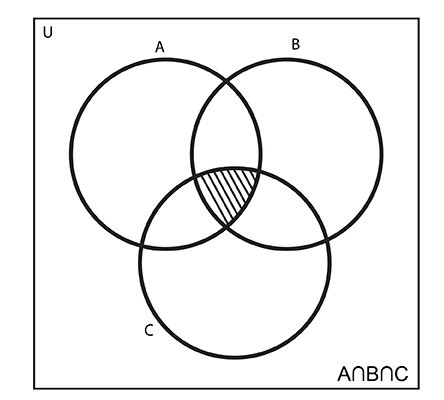# Python Set intersection()

The intersection() method returns a new set with elements that are common to all sets.

The intersection of two or more sets is the set of elements which are common to all sets. For example:

```A = {1, 2, 3, 4}
B = {2, 3, 4,  9}
C = {2, 4, 9 10}

Then,
A∩B = B∩A ={2, 3, 4}
A∩C = C∩A ={2, 4}
B∩C = C∩B ={2, 4, 9}

A∩B∩C = {2, 4}```The syntax of intersection() in Python is:

`A.intersection(*other_sets)`

## intersection() Parameters

The intersection() allows arbitrary number of arguments (sets).

Note: * is not part of the syntax. It is used to indicate that the method allows arbitrary number of arguments.

## Return Value from Intersection()

The intersection() method returns the intersection of set A with all the sets (passed as argument).

If argument is not passed to intersection(), it returns a shallow copy the set (A).

## Example 1: How intersection() works?

```A = {2, 3, 5, 4}
B = {2, 5, 100}
C = {2, 3, 8, 9, 10}

print(B.intersection(A))
print(B.intersection(C))
print(A.intersection(C))
print(C.intersection(A, B))
```

When you run the program, the output will be:

```{2, 5}
{2}
{2, 3}
{2}```

## More Examples

```A = {100, 7, 8}
B = {200, 4, 5}
C = {300, 2, 3}
D = {100, 200, 300}

print(A.intersection(D))
print(B.intersection(D))
print(C.intersection(D))
print(A.intersection(B, C, D))
```

When you run the program, the output will be:

```{100}
{200}
{300}
set()```

You can also find the intersection of sets using & operator

## Example 3: Set Intersection Using & operator

```A = {100, 7, 8}
B = {200, 4, 5}
C = {300, 2, 3, 7}
D = {100, 200, 300}

print(A & C)
print(A & D)

print(A & C & D)
print(A & B & C & D)
```

When you run the program, the output will be:

```{7}
{100}
set()
set()```